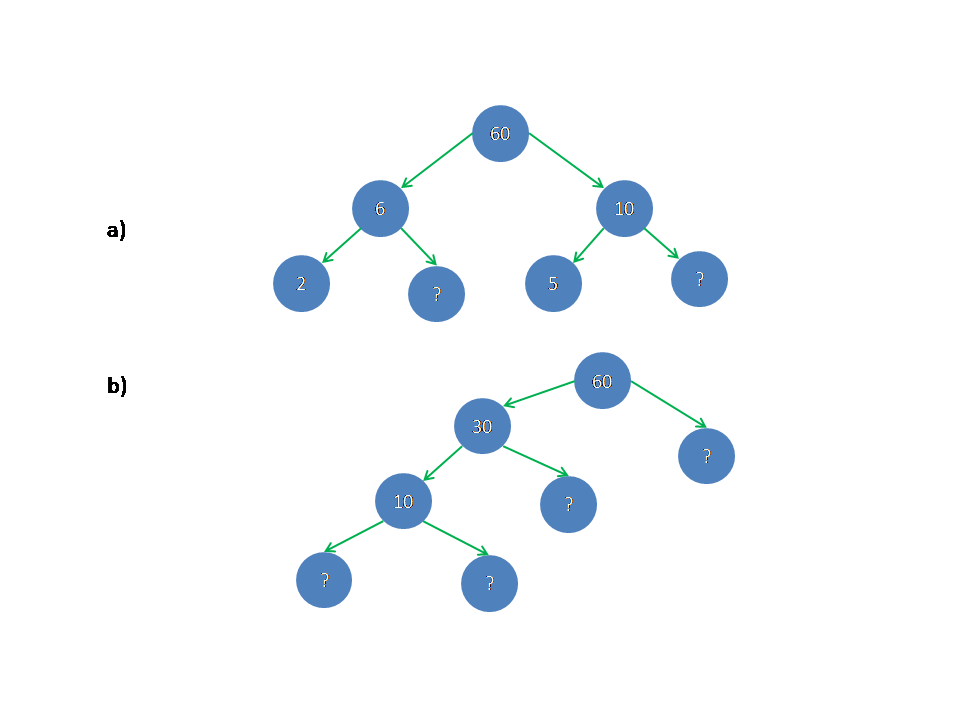## Ncert solutions for class 6th maths/chapter 3,Playing with Numbers solutions/factors

Ncert solutions for class 6th maths,playing with numbers,factors, chapter 3 Chapter 3 class 6 Playing with Numbers Maths Ncert solutions are given. First you study the textbook lesson very well. Observe and practice the example problems and solutions given in the textbook. 1. A factor of a number is an exact divisor of that number. …